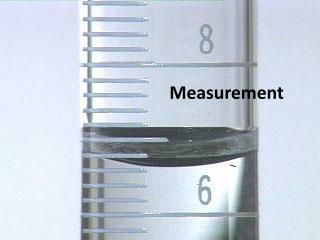DownloadDownload PresentationMeasurement

# Measurement

Download Presentation## Measurement

- - - - - - - - - - - - - - - - - - - - - - - - - - - E N D - - - - - - - - - - - - - - - - - - - - - - - - - - -
##### Presentation Transcript

1. Measurement

2. Measurements • A measurement is not complete unless it has a unit. A unit is the part of the measurement that tells us what scale or standard is being used to represent the results of the measurement. • The need for common units applies to scientists, who measure quantities such as mass, length, time, and temperature.

3. The Metric System • There are two measurement systems that are commonly used: • The English System (used in the United States) • The Metric System (used in most of the rest of the world) • In Chemistry, we will use the metric system. This system has long been preferred for most scientific work.

4. SI Units • The International System (or le SystemeInternationale in French) is the type of units that are based on the metric system. These units are also known as SI units. We will be using SI units in Chemistry. • SI Units

5. Metric Prefixes • The fundamental SI units are not always a convenient size, so the SI system uses prefixes to change the size of the unit.

6. Measuring Length • Length is measured with a ruler or meter stick • On a 12” ruler, there are 30.5cm • On a meter stick, there are 100cm • The SI unit is the meter, abbreviated “m”

7. Measuring Volume • Is the amount of three-dimensional space occupied by a substance. • The SI unit of volume is the cubic meter, abbreviated “m3,” but in Chemistry we will use liters, abbreviated “L” or the milliliter or abbreviated “mL” • Volume is measured with a graduated cylinder

8. Volume and the meniscus… • Measuring volume with a graduated cylinder is complicated by a meniscus. A meniscus is the curvature of the surface of the water. Water “sticks” to the walls of the graduated cylinder, but only on the sides and not the middle. When you look at the surface, the water level is not straight. Measurement should be at the lowest point. You must read the meniscus at eye level in order to get an accurate reading. You should place the graduated cylinder on the table and then lower your head to be able to read the meniscus at eye level.

9. Measuring Mass • The SI unit is the kilogram, abbreviated “kg,” but in Chemistry we will use grams, abbreviated “g” • Mass is measured with a balance

10. Measuring Temperature • The SI unit is the Kelvin, but in Chemistry we will use degrees Celsius, abbreviated, “°C” • Temperature is measured with a thermometer

11. Uncertainty in Measurement • A measurement always has some degree of uncertainty. • Whenever a measurement is made with a ruler, graduated cylinder, or thermometer, an estimate is required.

12. Uncertainty with a ruler • On the ruler to the right, you can accurately measure the pencil length to 8.2cm, but it is not exactly on the line. So, an estimation of the next division is required. This estimation is your degree of uncertainty. • One student might say 8.24cm, another might say 8.25cm, a third might say 8.23cm. • To account for the uncertainty, the measurement usually is written 8.24 ± 0.01 cm. The “± 0.01 cm” tells us that the measurement is uncertain to 1/100 of a centimeter.

13. Uncertainty with a thermometer • The same is true for thermometers: • On the thermometer to the right, you can accurately measure the temperature to be 23°C, but it is not exactly on the line. So an estimation of the next division is required. This estimation is your degree of uncertainty. • To account for the uncertainty, the measurement should be 23.5 ± 0.1 °C. This tells us that the thermometer is uncertain to 1/10 of a degree.

14. Uncertainty with a graduated cylinder • The same is true for graduated cylinders: • On the graduated cylinder to the right, you can accurately measure the temperature to be 43mL, but it is not exactly on the line. So an estimation of the next division is required. This estimation is your degree of uncertainty. • To account for the uncertainty, the measurement should be 43.1 ± 0.1 mL. This tells us that the graduated cylinder is uncertain to 1/10 of a degree.